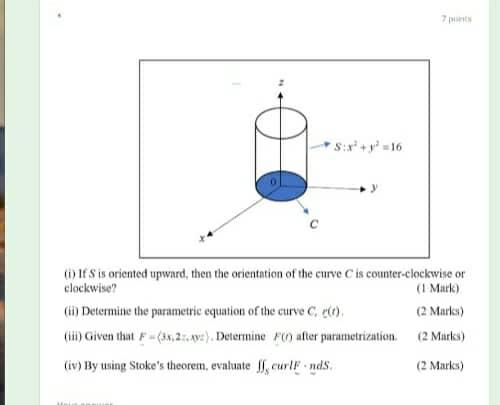### Create an Account

Already have account?

### Forgot Your Password ?

Home / Questions / Six + y = 16 If S is oriented upward, then the orientation of the curve C is counter-clock...

# Six + y = 16 If S is oriented upward, then the orientation of the curve C is counter-clockwise or clockwise? (1 Marlo) (6) Determine the parametric equation of the curve C) (2 Marks) coin) Given that

Six + y = 16 If S is oriented upward, then the orientation of the curve C is counter-clockwise or clockwise? (1 Marlo) (6) Determine the parametric equation of the curve C) (2 Marks) coin) Given that F(*.23.292) Determine after parametrization. (2 Marks) (iv) By using Stoke's theorem, evaluate curlFinds (2 Marks)Apr 14 2021 View more View Less

#### Answer (Solved)Subscribe To Get Solution